Example problem: The azimuthally Fourier-decomposed 3D Helmholtz equation and the use of perfectly matched layers

In this document we discuss the finite-element-based solution of the the Helmholtz equation in cylindrical polar coordinates, using a Fourier-decomposition of the solution in the azimuthal direction and with perfectly matched layers.

Compared to the Fourier-decomposed Helmholtz equation discussed in another tutorial, the formulation used here allows the imposition of the Sommerfeld radiation condition by means of so-called "perfectly matched layers" (PMLs) as an alternative to classical absorbing/approximate boundary conditions or DtN maps.

We start by reviewing the relevant theory and then present the solution of a simple model problem - the outward propagation of waves from the surface of a unit sphere.

 AcknowledgementsThis tutorial and the associated driver codes were developed jointly with Matthew Walker (The University of Manchester), with financial support from Thales Underwater Ltd.

# Theory: The azimuthally Fourier-decomposed Helmholtz equation

The Helmholtz equation governs time-harmonic solutions of problems governed by the linear wave equation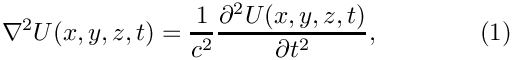where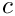is the wavespeed. Assuming that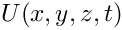is time-harmonic, with frequency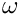, we write the real functionas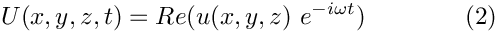where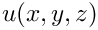is complex-valued. This transforms (1) into the Helmholtz equation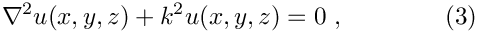where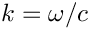is the wavenumber. Like other elliptic PDEs the Helmholtz equation admits Dirichlet, Neumann (flux) and Robin boundary conditions.

If the equation is solved in an unbounded spatial domain (e.g. in scattering problems) the solution must also satisfy the so-called Sommerfeld radiation condition, which in 3D has the form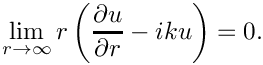Mathematically, this condition is required to ensure the uniqueness of the solution (and hence the well-posedness of the problem). In a physical context, such as a scattering problem, the condition ensures that scattering of an incoming wave only produces outgoing not incoming waves from infinity.

These equations can be solved using oomph-lib's cartesian Helmholtz elements, described in
another tutorial. Here we consider an alternative approach in which we solve the equations in cylindrical polar coordinates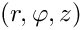, related to the cartesian coordinates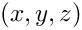via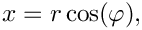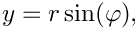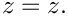We then decompose the solution into its Fourier components by writing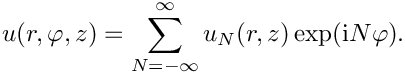Since the governing equations are linear we can compute each Fourier component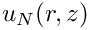individually by solving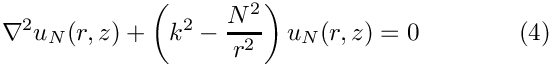while specifying the Fourier wavenumber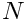as a parameter.

# Discretisation by finite elements

The discretisation of the Fourier-decomposed Helmholtz equation itself only requires a trivial modification of its cartesian counterpart. Since most practical applications of the Helmholtz equation involve complex-valued solutions, we provide separate storage for the real and imaginary parts of the solution – each Node therefore stores two unknowns values. By default,the real and imaginary parts are stored as values 0 and 1, respectively;

The application of Dirichlet and Neumann boundary conditions is straightforward and follows the pattern employed for the solution of the Poisson equation:

• Dirichlet conditions are imposed by pinning the relevant nodal values and setting them to the appropriate prescribed values.
• Neumann (flux) boundary conditions are imposed via FaceElements (here the PMLFourierDecomposedHelmholtzFluxElements). As usual we attach these to the faces of the "bulk" elements that are subject to the Neumann boundary conditions.

The imposition of the Sommerfeld radiation condition for problems in infinite domains is slightly more complicated. In the next section we will discuss a method of representing the Sommerfeld radiation condition numerically by means of perfectly matched layers.

# Perfectly matched layers

The idea behind perfectly matched layers is illustrated in the figure below. The actual physical/mathematical problem has to be solved in the infinite domain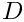(shown on the left), with the Sommerfeld radiation condition ensuring the suitable decay of the solution at large distances from the region of interest (the vicinity of the scatterer, say).

If computations are performed in a finite computational domain,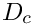, (shown in the middle), spurious wave reflections are likely to be generated at the artificial boundary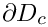of the computational domain.

The idea behind PML methods is to surround the actual computational domainwith a layer of "absorbing" material whose properties are chosen such that the outgoing waves are absorbed within it, without creating any artificial reflected waves at the interface between the PML layer and the computational domain.

Our implementation of the perfectly matched layers follows the development in A. Bermudez, L. Hervella-Nieto, A. Prieto, and R. Rodriguez "An optimal perfectly matched layer with unbounded absorbing function for time-harmonic acoustic scattering problems" Journal of Computational Physics 223 469-488 (2007) and we assume the boundaries of the computational domain to be aligned with the coordinate axes, as shown in the sketch below.

The method requires a slight further generalisation of the equations, achieved by introducing the complex coordinate mappingwithin the perfectly matched layers. The choice of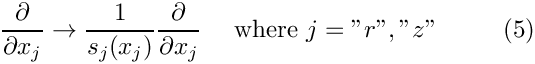and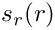depends on the orientation of the PML layer. Since we are restricting ourselves to axis-aligned mesh boundaries we distinguish three different cases

• For layers that are aligned with the r axis (such as the top and bottom PML layers) we set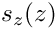where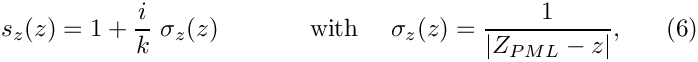is the z-coordinate of the outer boundary of the PML layer, and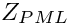• For the right layer that is aligned with the z axis we set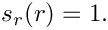and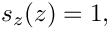where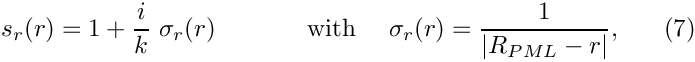is the r-coordinate of the outer boundary of the PML layer.
• In corner regions that are bounded by two axis-aligned PML layers (with outer coordinatesand) we set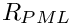and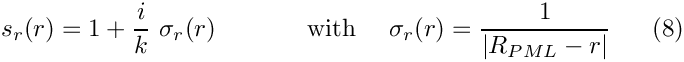• Finally, in the actual computational domain (outside the PML layers) we set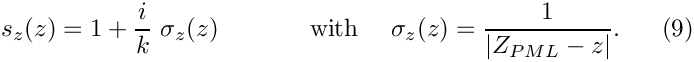# Implementation within oomph-lib

The finite-element-discretised equations (modified by the PML terms discussed above) are implemented in the PMLFourierDecomposedHelmholtzEquations class. As usual, we provide fully functional elements by combining these with geometric finite elements (from the Q and T families – corresponding (in 2D) to triangles and quad elements). By default, the PML modifications are disabled, i.e.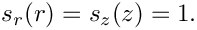andare both set to 1.

The generation of suitable 2D PML meshes along the axis-aligned boundaries of a given bulk mesh is facilitated by helper functions which automatically erect layers of (quadrilateral) PML elements. The layers are built from QPMLFourierDecomposedHelmholtzElement<NNODE_1D> elements and the parameter NNODE_1D is automatically chosen to match that of the elements in the bulk mesh. The bulk mesh can contain quads or triangles (as shown in the specific example presented below).

# A specific example: Outward propagation of waves from the surface of an oscillating sphere

We will now demonstrate the methodology for a specific example: the propagation of waves from the surface of a unit sphere.

The specific domain used in this case can be seen in the figure below. We create an unstructured mesh of six-noded TPMLFourierDecomposedHelmholtzElements to create the finite computational domain surrounding a sphere. This is surrounded by three axis-aligned PML layers and two corner meshes (each made of nine-noded QPMLFourierDecomposedHelmholtzElements).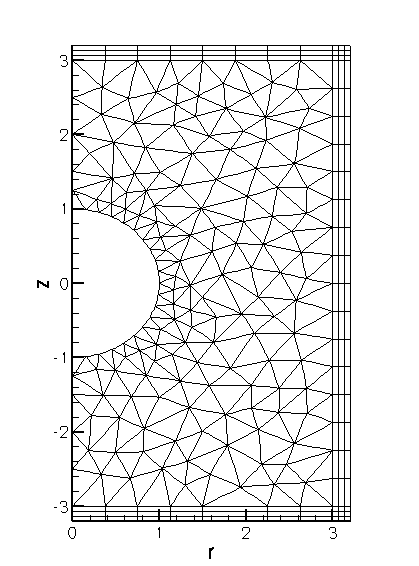The computational domain used in the example problem.

We construct an exact solution to the problem by applying Neumann/flux boundary condition on the inner spherical boundary such that the imposed fluxis consistent with the exact solution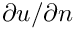in spherical polar coordinates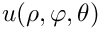, given by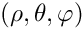where the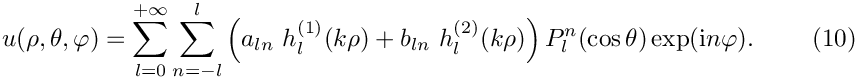are arbitrary coefficients and the functions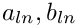are the spherical Hankel functions of first and second kind, respectively, expressed in terms the spherical Bessel functions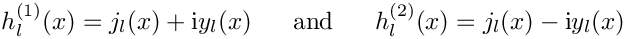The functions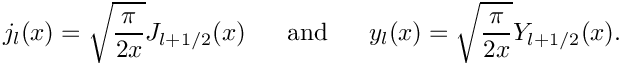are the associated Legendre functions, expressed in terms of the Legendre polynomials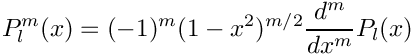This definition shows that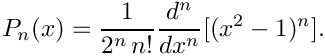for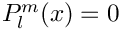which explains the limited range of summation indices in the second sum in (10).

The relation between the cylindrical polar coordinates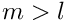and spherical polar coordinatesis given by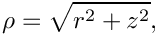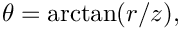so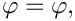remains unchanged, and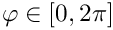sweeps from the north pole (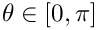), via the equator (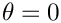) to the south pole (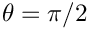).

# Results

The two figures below show a comparison between the computed and exact solutions for a Fourier wavenumber of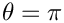, wavenumber squared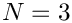.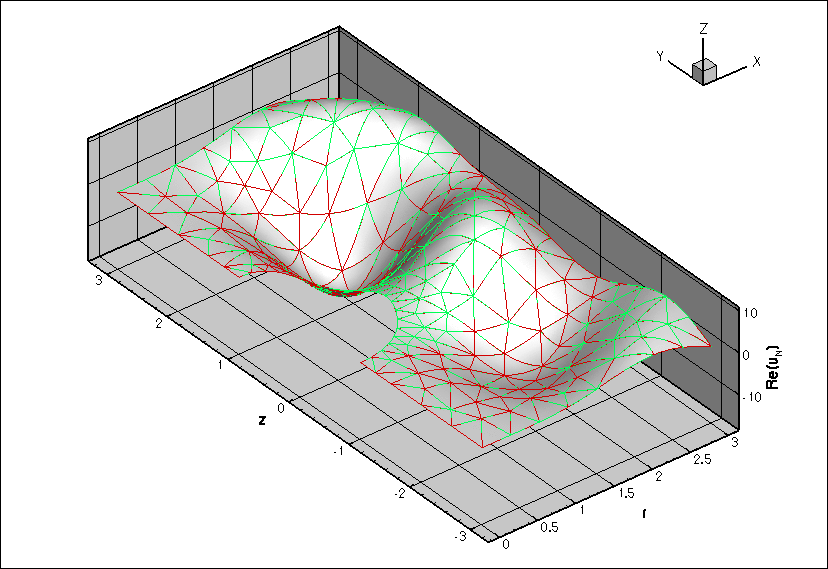Plot of the computed (red) and exact (green) real parts of the solution of the Fourier-decomposed Helmholtz equation.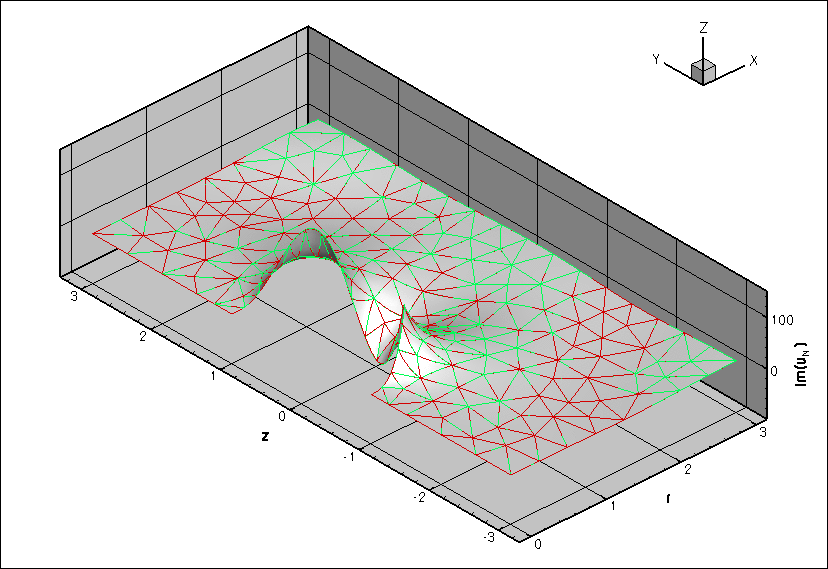Plot of the computed (red) and exact (green) imaginary parts of the solution of the Fourier-decomposed Helmholtz equation.

# The numerical solution

## The global namespace

As usual, we define the problem parameters in a global namespace. The main parameters are the wavenumber squared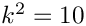, the PML thickness, the number of elements within the PML layer, and the Fourier wavenumber.

//===== start_of_namespace=============================================
/// Namespace for the Fourier decomposed Helmholtz problem parameters
//=====================================================================
{
/// Output directory
string Directory="RESLT";
/// Frequency
double K_squared = 10.0;
/// Default physical PML thickness
double PML_thickness=4.0;
/// Default number of elements within PMLs
unsigned Nel_pml=15;
/// Target area for initial mesh
double Element_area = 0.1;
/// The default Fourier wave number
int N_fourier=0;
////////////////////////////////////////////////////////////////// //////////////////////////////////...
string Directory
Output directory.
double K_squared
Frequency.
int N_fourier
The default Fourier wave number.
double Element_area
Target area for initial mesh.
double PML_thickness
Default physical PML thickness.
unsigned Nel_pml
Default number of elements within PMLs.

Next we define the coefficients

/// Number of terms in the exact solution
unsigned N_terms=6;
/// Coefficients in the exact solution
Vector<double> Coeff(N_terms,1.0);
unsigned N_terms
Number of terms in the exact solution.
Vector< double > Coeff(N_terms, 1.0)
Coefficients in the exact solution.

required for the specification of the exact solution

/// Exact solution as a Vector of size 2, containing real and imag parts
void get_exact_u(const Vector<double>& x, Vector<double>& u)
void get_exact_u(const Vector< double > &x, Vector< double > &u)
Exact solution as a Vector of size 2, containing real and imag parts.

and its derivative

/// Get -du/dr (spherical r) for exact solution. Equal to prescribed
/// flux on inner boundary.
void exact_minus_dudr(const Vector<double>& x, std::complex<double>& flux)
void exact_minus_dudr(const Vector< double > &x, std::complex< double > &flux)
Get -du/dr (spherical r) for exact solution. Equal to prescribed flux on inner boundary.

whose listings we omit here.

## The driver code

The driver code is very straightforward. We create the problem object,

//===== start_of_main=====================================================
/// Driver code for Pml Fourier decomposed Helmholtz problem
//========================================================================
int main(int argc, char **argv)
{
int main(int argc, char **argv)
Driver code for Pml Fourier decomposed Helmholtz problem.
// Create the problem with 2D six-node elements from the
// TPMLFourierDecomposedHelmholtzElement family.
<TPMLFourierDecomposedHelmholtzElement<3> >
problem;
////////////////////////////////////////////////////////////////// //////////////////////////////////...

and define the output directory.

// Create label for output
DocInfo doc_info;
// Set output directory
doc_info.set_directory(ProblemParameters::Directory);

Finally, we solve the problem and document the results.

// Solve the problem with Newton's method
problem.newton_solve();
//Output the solution
problem.doc_solution(doc_info);
} //end of main

## The problem class

The problem class is very similar to that employed for the solution of the 2D Helmholtz equation with flux boundary conditions. We provide helper functions to create the PML meshes and to apply the boundary conditions (mainly because these tasks have to be performed repeatedly in the spatially adaptive version of this code which is not discussed explicitly here; but see the exercise on Spatial adaptivity).

//========= start_of_problem_class=====================================
/// Problem class
//=====================================================================
template<class ELEMENT>
{
public:
/// Constructor
/// Destructor (empty)
/// Update the problem specs before solve (empty)
/// Update the problem after solve (empty)
/// Doc the solution. DocInfo object stores flags/labels for where the
/// output gets written to
void doc_solution(DocInfo& doc_info);
/// Create PML meshes
/// Create mesh of face elements that monitor the radiated power
void actions_after_newton_solve()
Update the problem after solve (empty)
void create_power_monitor_mesh()
Create mesh of face elements that monitor the radiated power.
~PMLFourierDecomposedHelmholtzProblem()
Destructor (empty)
void create_pml_meshes()
Create PML meshes.
void actions_before_newton_solve()
Update the problem specs before solve (empty)
void doc_solution(DocInfo &doc_info)
Doc the solution. DocInfo object stores flags/labels for where the output gets written to.

The private member data includes pointers to the bulk mesh,

/// Pointer to the "bulk" mesh
TriangleMesh<ELEMENT>* Bulk_mesh_pt;

a pointer to the mesh of FaceElements that apply the flux boundary condition on the surface of the sphere,

/// Mesh of FaceElements that apply the flux bc on the inner boundary
Mesh* Helmholtz_inner_boundary_mesh_pt;

and the various PML sub-meshes:

/// Pointer to the right PML mesh
Mesh* PML_right_mesh_pt;
/// Pointer to the top PML mesh
Mesh* PML_top_mesh_pt;
/// Pointer to the bottom PML mesh
Mesh* PML_bottom_mesh_pt;
/// Pointer to the top right corner PML mesh
Mesh* PML_top_right_mesh_pt;
/// Pointer to the bottom right corner PML mesh
Mesh* PML_bottom_right_mesh_pt;
/// Trace file
ofstream Trace_file;
}; // end of problem class

## The problem constructor

We open a trace file in which we record the radiated power and create the Circle object that defines the curvilinear inner boundary of the domain.

//=======start_of_constructor=============================================
/// Constructor for Pml Fourier-decomposed Helmholtz problem
//========================================================================
template<class ELEMENT>
{
string trace_file_location = ProblemParameters::Directory + "/trace.dat";
// Open trace file
Trace_file.open(trace_file_location.c_str());
/// Setup "bulk" mesh
// Create the circle that represents the inner boundary
double x_c=0.0;
double y_c=0.0;
double r_min=1.0;
Circle* inner_circle_pt=new Circle(x_c,y_c,r_min);

Next we specify the the outer radius of computational domain

double r_max=3.0;

and define its polygonal outer boundary:

// Edges/boundary segments making up outer boundary
//-------------------------------------------------
Vector<TriangleMeshCurveSection*> outer_boundary_line_pt(6);
// All poly boundaries are defined by two vertices
Vector<Vector<double> > boundary_vertices(2);
// Bottom straight boundary on symmetry line
//------------------------------------------
boundary_vertices.resize(2);
boundary_vertices=0.0;
boundary_vertices=-r_min;
boundary_vertices.resize(2);
boundary_vertices=0.0;
boundary_vertices=-r_max;
unsigned boundary_id=0;
outer_boundary_line_pt=
new TriangleMeshPolyLine(boundary_vertices,boundary_id);

Next we define the curvilinear inner boundary in terms of a TriangleMeshCurviLine which defines the surface of the sphere,

// Inner circular boundary:
//-------------------------
// Number of segments used for representing the curvilinear boundary
unsigned n_segments = 20;
// The intrinsic coordinates for the beginning and end of the curve
double s_start = 0.5*MathematicalConstants::Pi;
double s_end = -0.5*MathematicalConstants::Pi;
boundary_id = 5;
outer_boundary_line_pt=
new TriangleMeshCurviLine(inner_circle_pt,
s_start,
s_end,
n_segments,
boundary_id);

and combine the various pieces of the boundary to the closed outer boundary:

// Create closed curve that defines outer boundary
//------------------------------------------------
TriangleMeshClosedCurve *outer_boundary_pt =
new TriangleMeshClosedCurve(outer_boundary_line_pt);

Finally, we specify the mesh parameters,

// Use the TriangleMeshParameters object for helping on the manage of the
// TriangleMesh parameters. The only parameter that needs to take is the
// outer boundary.
TriangleMeshParameters triangle_mesh_parameters(outer_boundary_pt);
// Specify maximum element area
double element_area = ProblemParameters::Element_area;
triangle_mesh_parameters.element_area() = element_area;

build the bulk mesh, and add it to the problem:

// Create the bulk mesh
Bulk_mesh_pt= new TriangleMesh<ELEMENT>(triangle_mesh_parameters);
// Add the bulk mesh to the problem

Next, we create the FaceElements that apply the flux boundary condition on the boundary of the sphere and add the corresponding mesh to the problem too:

// Create flux elements on inner boundary
Helmholtz_inner_boundary_mesh_pt=new Mesh;
create_flux_elements_on_inner_boundary();
// ...and add the mesh to the problem

We create another set of FaceElements that allow the computation of the radiated flux over the outer boundaries of the domain:

// Attach the power monitor elements
Power_monitor_mesh_pt=new Mesh;
create_power_monitor_mesh();

(This mesh does not need to be added to the problem since its elements merely act as post-processing tools and do not provide any contributions to the problem's residual vector.

We build the PML meshes and combine the various sub-meshes to the problem's global mesh:

// Create the pml meshes
create_pml_meshes();
// Build the Problem's global mesh from its various sub-meshes
build_global_mesh();

We complete the problem setup by passing the problem parameters to the elements, using the helper function complete_problem_setup() (Remember that even the elements in the PML layers need to be told about these parameters since they adjust theandfunctions in terms of these parameters).

// Complete the build of all elements
complete_problem_setup();

Finally we assign the equation numbers,

// Setup equation numbering scheme
cout <<"Number of equations: " << assign_eqn_numbers() << std::endl;

The problem can now be solved.

## Impose flux on inner boundary

The function create_flux_elements() creates the FaceElements required to apply the flux/Neumann boundary conditions on the boundary of the sphere.

//============start_of_create_flux_elements=================
/// Create flux elements on inner boundary
//==========================================================
template<class ELEMENT>
{
// Apply flux bc on inner boundary (boundary 5)
unsigned b=5;
// Loop over the bulk elements adjacent to boundary b
unsigned n_element = Bulk_mesh_pt->nboundary_element(b);
for(unsigned e=0;e<n_element;e++)
{
// Get pointer to the bulk element that is adjacent to boundary b
ELEMENT* bulk_elem_pt = dynamic_cast<ELEMENT*>(
Bulk_mesh_pt->boundary_element_pt(b,e));
//Find the index of the face of element e along boundary b
int face_index = Bulk_mesh_pt->face_index_at_boundary(b,e);
// Build the corresponding prescribed incoming-flux element
PMLFourierDecomposedHelmholtzFluxElement<ELEMENT>*
flux_element_pt = new
PMLFourierDecomposedHelmholtzFluxElement<ELEMENT>
(bulk_elem_pt,face_index);
//Add the prescribed incoming-flux element to the surface mesh
// Set the pointer to the prescribed flux function
flux_element_pt->flux_fct_pt() = &ProblemParameters::exact_minus_dudr;
} //end of loop over bulk elements adjacent to boundary b
} // end of create flux elements on inner boundary
void create_flux_elements_on_inner_boundary()
Create flux elements on inner boundary.

## Create power monitor mesh

The function create_power_monitor_mesh creates the FaceElements that allow the computation of the radiated power over the outer boundary of the computational domain.

//===================start_of_create_power_monitor_mesh===================
/// Create BC elements on outer boundary
//========================================================================
template<class ELEMENT>
{
// Loop over outer boundaries
for (unsigned b=1;b<4;b++)
{
// Loop over the bulk elements adjacent to boundary b?
unsigned n_element = Bulk_mesh_pt->nboundary_element(b);
for(unsigned e=0;e<n_element;e++)
{
// Get pointer to the bulk element that is adjacent to boundary b
ELEMENT* bulk_elem_pt = dynamic_cast<ELEMENT*>(
Bulk_mesh_pt->boundary_element_pt(b,e));
//Find the index of the face of element e along boundary b
int face_index = Bulk_mesh_pt->face_index_at_boundary(b,e);
// Build the corresponding element
PMLFourierDecomposedHelmholtzPowerMonitorElement<ELEMENT>*
flux_element_pt = new
PMLFourierDecomposedHelmholtzPowerMonitorElement<ELEMENT>
(bulk_elem_pt,face_index);
}
}
} // end of create_power_monitor_mesh

## Complete problem setup

The helper function complete_problem_setup() completes the setup of the elements by passing pointers to the relevant problem parameters to them. We apply zero Dirichlet boundary conditions on the centreline if the Fourier wavenumber is odd.

//=================start_of_complete_problem_setup==================
// Complete the build of all elements so that they are fully
// functional
//==================================================================
template<class ELEMENT>
{
// Complete the build of all elements so they are fully functional
unsigned n_element = this->mesh_pt()->nelement();
for(unsigned i=0;i<n_element;i++)
{
// Upcast from GeneralsedElement to the present element
PMLFourierDecomposedHelmholtzEquations *el_pt = dynamic_cast<
PMLFourierDecomposedHelmholtzEquations*>(
mesh_pt()->element_pt(i));
if (!(el_pt==0))
{
//Set the frequency pointer
el_pt->k_squared_pt()=&ProblemParameters::K_squared;
// Set pointer to Fourier wave number
el_pt->pml_fourier_wavenumber_pt()=&ProblemParameters::N_fourier;
}
}
// If the Fourier wavenumber is odd, then apply zero dirichlet boundary
// conditions on the two straight boundaries on the symmetry line.
{
cout
<< "Zero Dirichlet boundary condition has been applied on symmetry line\n";
cout << "due to an odd Fourier wavenumber\n" << std::endl;
apply_zero_dirichlet_boundary_conditions();
}
} // end of complete_problem_setup

## Apply zero Dirichlet boundary conditions

This final helper function pins both nodal values (representing the real and imaginary part of the solution) on the centreline and sets their values to zero.

//=========start_of_apply_zero_dirichlet_boundary_conditions========
// Apply extra bounday conditions if given an odd Fourier wavenumber
//==================================================================
template<class ELEMENT>
{
// Apply zero dirichlet conditions on the bottom straight boundary
// and the top straight boundary located on the symmetry line.
// Bottom straight boundary on symmetry line:
{
//Boundary id
unsigned b=0;
// How many nodes are there?
unsigned n_node=Bulk_mesh_pt->nboundary_node(b);
for (unsigned n=0;n<n_node;n++)
{
// Get the node
Node* nod_pt=Bulk_mesh_pt->boundary_node_pt(b,n);
// Pin the node
nod_pt->pin(0);
nod_pt->pin(1);
// Set the node's value
nod_pt->set_value(0, 0.0);
nod_pt->set_value(1, 0.0);
}
}
// Top straight boundary on symmetry line:
{
//Boundary id
unsigned b=4;
// How many nodes are there?
unsigned n_node=Bulk_mesh_pt->nboundary_node(b);
for (unsigned n=0;n<n_node;n++)
{
// Get the node
Node* nod_pt=Bulk_mesh_pt->boundary_node_pt(b,n);
// Pin the node
nod_pt->pin(0);
nod_pt->pin(1);
// Set the node's value
nod_pt->set_value(0, 0.0);
nod_pt->set_value(1, 0.0);
}
}
} // end of apply_zero_dirichlet_boundary_conditions

## Post-processing

The post-processing function doc_solution(...) outputs the solution within the bulk, the solution within the PMLs, the exact solution and the radiated power

//===============start_of_doc=============================================
/// Doc the solution: doc_info contains labels/output directory etc.
//========================================================================
template<class ELEMENT>
doc_solution(DocInfo& doc_info)
{
ofstream some_file;
char filename;
// Number of plot points: npts x npts
unsigned npts=5;
// Output solution within the bulk mesh
//-------------------------------------
sprintf(filename,"%s/soln%i.dat",doc_info.directory().c_str(),
doc_info.number());
some_file.open(filename);
Bulk_mesh_pt->output(some_file,npts);
some_file.close();
// Output solution within pml domains
//-----------------------------------
sprintf(filename,"%s/pml_soln%i.dat",doc_info.directory().c_str(),
doc_info.number());
some_file.open(filename);
PML_top_mesh_pt->output(some_file,npts);
PML_right_mesh_pt->output(some_file,npts);
PML_bottom_mesh_pt->output(some_file,npts);
PML_top_right_mesh_pt->output(some_file,npts);
PML_bottom_right_mesh_pt->output(some_file,npts);
some_file.close();
// Output exact solution
//----------------------
sprintf(filename,"%s/exact_soln%i.dat",doc_info.directory().c_str(),
doc_info.number());
some_file.open(filename);
Bulk_mesh_pt->output_fct(some_file,npts,ProblemParameters::get_exact_u);
some_file.close();
double power=0.0;
//----------------------------------
sprintf(filename,"%s/power%i.dat",doc_info.directory().c_str(),
doc_info.number());
some_file.open(filename);
// Accumulate contribution from elements
unsigned nn_element=Power_monitor_mesh_pt->nelement();
for(unsigned e=0;e<nn_element;e++)
{
PMLFourierDecomposedHelmholtzPowerMonitorElement<ELEMENT> *el_pt =
dynamic_cast<PMLFourierDecomposedHelmholtzPowerMonitorElement
<ELEMENT>*>(Power_monitor_mesh_pt->element_pt(e));
power += el_pt->global_power_contribution(some_file);
}
some_file.close();
oomph_info << "Total radiated power: " << power << std::endl;

## The enumeration of the unknowns

As discussed in the introduction, most practically relevant solutions of the Helmholtz equation are complex valued. Since oomph-lib's solvers only deal with real (double precision) unknowns, the equations are separated into their real and imaginary parts. In the implementation of the Helmholtz elements, we store the real and imaginary parts of the solution as two separate values at each node. By default, the real and imaginary parts are accessible via Node::value(0) and Node::value(1). However, to facilitate the use of the elements in multi-physics problems we avoid accessing the unknowns directly in this manner but provide the virtual function

std::complex<unsigned> PMLFourierDecomposedHelmholtzEquations::u_index_pml_fourier_decomposed_helmholtz()

which returns a complex number made of the two unsigneds that indicate which nodal value represents the real and imaginary parts of the solution. This function may be overloaded in combined multi-physics elements in which a Helmholtz element is combined (by multiple inheritance) with another element, using the strategy described in the Boussinesq convection tutorial.

## PML damping functions

The choice for the absorbing functions in our implementation of the PMLs is not unique. There are alternatives varying in both order and continuity properties. The current form is the result of several feasibility studies and comparisons found in both Bermudez et al. These damping functions produce an acceptable result in most practical situations without further modifications. For very specific applications, alternatives may need to be used and can easily be implemented by constructing a PML Mapping class and passing a pointer to the elements.

## Exercises

### Changing the Fourier wavenumber

The generalised Fourier-decomposed Helmholtz equation allows for various Fourier wavenumbers. Confirm that a zero Dirichlet boundary condition is applied to odd Fourier wavenumbers.

### Comparison of results

Compare the results computed by the current driver code against those obtained when the Sommerfeld radiation condition is imposed by a DtN mapping, as discussed in another tutorial.

### Changing perfectly matched layer parameters

Confirm that only a very small number of PML elements (across the thickness of the PML layer) is required to effectively damp the outgoing waves. Explore the effects of altering the number of elements layer while keeping the PML thickness constant.

A second parameter that can be adjusted is the geometrical thickness of the perfectly matched layers. Explore the effects of altering the thickness while maintaining the number of elements within the PML layer.

### Large wavenumbers

For Helmholtz problems in general, ill-conditioning appears as the wavenumber becomes very large. By altering, explore the limitations of both the mesh and the solver in terms of this parameter. Try adjusting the target element size in order to alleviate resolution-related effects. Assess the effectiveness of the perfectly matched layers in high wavenumber problems.

The driver code discussed above already contains the straightforward modifications required to enable spatial adaptivity. Explore this (by recompiling the code with -DADAPTIVE). You will note that the driver code for this case is modified slightly – the system is no longer driven by flux boundary conditions on the boundary of the sphere, but by a point source inside the domain. This was done to demonstrate the advantage of spatial adaptivity for such problems. The benefits of spatial adaptation in problems without any singularities tends to be limited since Helmholtz (and most other wave-type problems) require fairly uniform meshes throughout the domain.

### Default values for problem parameters

Following our usual convention, we provide default values for problem parameters where this is sensible. For instance, if the pointer to the PML damping class is not set, it will default to the best known PML mapping function proposed by Bermudez et al. Some parameters, such as the wavenumber squared, do need to be set since there are no obvious defaults. If oomph-lib is compiled in PARANOID mode, an error is thrown if the relevant pointers haven't been set. Without paranoia, you get a segmentation fault...

Confirm that this is the case by commenting out the relevant assignments.